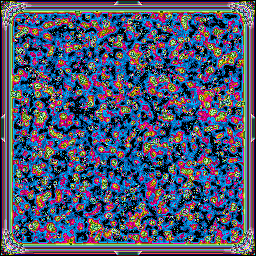• Home
• Files
• The true spirit of delight, the exaltation, the sense of being more than Man, which is the touchstone of the highest excellence, is to be found in mathematics as surely as in poetry.

# Day 10

Previous: Day 09 | Next: Day 11
###AUTO-ACQUIRED DATA FOLLOWS…
archivist/day10.lisp
archivist/day10.lisp
```(asdf:load-system :split-sequence)
(defpackage \#:aoc-10
(:use \#:cl \#:split-sequence \#:iterate))
(in-package \#:aoc-10)

(defun getinput ()
(mapcar (lambda (x)
(parse-integer x))

(defun part1 ()
(let\* ((input (sort (getinput) \#&\#39;<))
(proc
(mapcar (lambda (x y)
(- y x))
(cons 0 (subseq input 0 (length input) ))
(nreverse
(cons (+ (car (last input)) 3)
(nreverse input) )))))
(\* (count 1 proc)
(count 3 proc))))

(defun part2-walk (l cache)
1
(reduce \#&\#39;+
(mapcar
(lambda (remain)
(if (and
(car remain)
(<= (- (car remain) (car l)) 3))
(multiple-value-bind
(result exists)
(gethash (car remain) cache)
(if exists result
(setf (gethash (car remain) cache)
(part2-walk remain cache))))
0))
`(,(cdr l) ,(cddr l) ,(cdddr l))))))

(defun part2-slow ()
(let\* ((input (cons 0
(append (sort (getinput) \#&\#39;<)
(list
(+ 3
(apply \#&\#39;max
(getinput)))))))
(mycache (make-hash-table :test \#&\#39;equal)))
(part2-walk input mycache)))

(defun validp (a b)
(<= (- b a) 3))

(defun getif (val later)
(if (validp val (car later))
0))

(defun part2 ()
(let\* ((input (nreverse (cons 0 (sort (getinput) \#&\#39;<))))
(tri (list (pop input) 1))
(two (list (pop input) 1))
(one-f (pop input))
(one (list one-f
(+ (getif one-f two)
(getif one-f tri)))))
(iterate (while input)
(let\* ((newval (pop input))
(new (list newval
(reduce \#&\#39;+
(mapcar
(lambda (x)
(getif newval x))
(list tri two one))))))
(setf tri (copy-list two))
(setf two (copy-list one))
(setf one (copy-list new))))
```
day-10/day10.lisp
day-10/day10.lisp
```(defun load-nums (filename)
(with-open-file (stream filename)
(loop for line = (read-line stream nil)
while line
collect (parse-integer line))))

(defun differences (nums)
(if (cdr nums) ; length >= 2
(cons
(differences (cdr nums)))))

(defun find-paths (remaining-nodes alist)
; alist stores number of paths from some nodes
(let\* ((current-node (car remaining-nodes))
(options (list
(assoc (+ current-node 1) alist)
(assoc (+ current-node 2) alist)
(assoc (+ current-node 3) alist)))) ; some options may be nil
(let\* ((path-sum (reduce &\#39;+ (mapcar (lambda (x) (if (null x) 0 (cdr x))) options)))
(paths (if (= 0 path-sum)
1 ; this must be the first go-through
path-sum)))
(if (= current-node 0)
paths
(find-paths (cdr remaining-nodes) (acons current-node paths alist))))))

(let\* ((input-nums (sort (load-nums "input10.txt") &\#39;>))
(lowest-num 0) (highest-num (+ 3 (car input-nums)))
(all-nums (append (list highest-num) input-nums (list lowest-num))))
(let ((diffs (differences all-nums)))
(format t "~a~%"
(\* (count-if (lambda (x) (= x 3)) diffs)
(count-if (lambda (x) (= x 1)) diffs))))
(format t "~a~%"
(find-paths all-nums &\#39;())))
```
javanon/day10.js
javanon/day10.js
```dataset = document.querySelector("body > pre");
numbers = dataset.innerText.trim().split("\n").map(val => Number(val)).sort( (a, b) => a - b);

function day10\_partA (input) {
var counts = input.map( (v, i, arr) => v - (arr[i - 1] || 1))
.reduce( (acc, item) => {
acc[item]++;
return acc;
}, {1: 1, 3: 1});
return counts \* counts;
}

function day10\_partB (input) {
var sums = {0: 1};
input.forEach(item => sums[item] = (sums[item - 3] || 0) + (sums[item - 2] || 0) + (sums[item - 1] || 0));
return sums[input[input.length - 1]];
}
```
steveklabnik/steveklabnik-day10.rs
steveklabnik/steveklabnik-day10.rs
```fn solve(input: &str) -> (u32, u64) {
let mut adapters: Vec<usize> = input.lines().map(|s| s.parse().unwrap()).collect();
let max = adapters.last().unwrap() + 3;

let mut last = 0;
let mut count = vec![0; max + 1];
count = 1;

.iter()
.inspect(|&&n| {
count[n] = (1..=3)
.filter\_map(|d| n.checked\_sub(d).map(|n| count[n]))
.sum()
})
.map(|&n| n - std::mem::replace(&mut last, n))
.fold((0, 0), |(a, b), d| {
(a + u32::from(d == 1), b + u32::from(d == 3))
});

(a.0 \* a.1, count[max])
}
```

Tags: